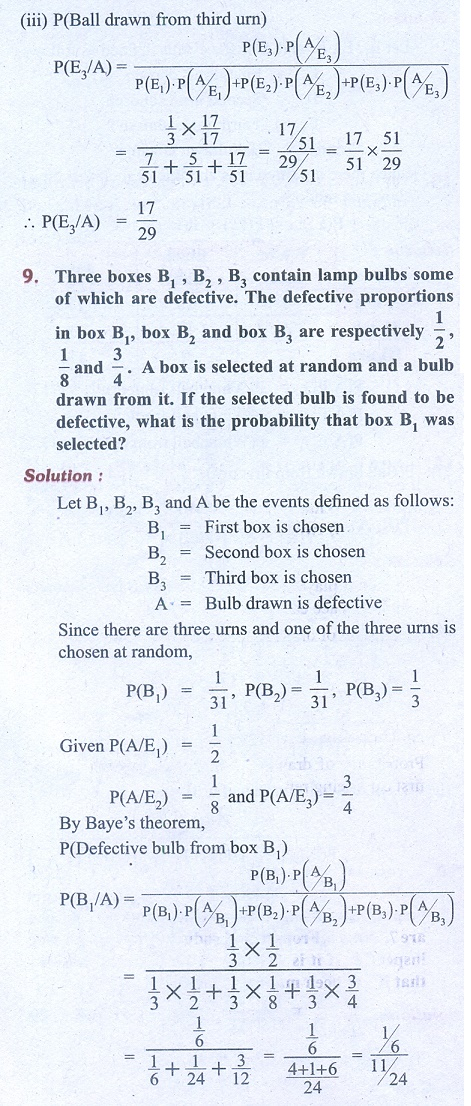Home | | Business Maths 11th std | Exercise 8.2: Baye‚Äôs Theorem

# Exercise 8.2: Baye‚Äôs Theorem

Business Mathematics and Statistics Book back answers and solution for Exercise questions - Descriptive statistics and probability: Baye‚Äôs TheoremTags : Problem Questions with Answer, Solution | Descriptive statistics and probability , 11th Business Mathematics and Statistics(EMS) : Chapter 8 : Descriptive statistics and probability
Study Material, Lecturing Notes, Assignment, Reference, Wiki description explanation, brief detail
11th Business Mathematics and Statistics(EMS) : Chapter 8 : Descriptive statistics and probability : Exercise 8.2: Baye‚Äôs Theorem | Problem Questions with Answer, Solution | Descriptive statistics and probability